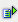The function quotient (num, divisor) returns the whole-numbered part of a division.

Syntax: =quotient(num,divisor)Example =quotient(0.3) is 0 =quotient(1.3) is 0 =quotient(2.3) is 0 =quotient(3.3) is 1 =quotient(4,3) is 1